• 精品文档助力人生欢迎关注小编 通过共享单车分析共享经济的本质及前景展望 谭顺文 摘 要共享单车以其便捷性在我国各大城市受到群众的热烈欢迎为人们节省了不少的出行成本在共享单车飞速发展的背后是共享经济理论的...
• 一、提出问题在本项目中，参与者被要求将历史使用模式与天气...本次共享单车数据分析项目数据源于Kaggle。获取数据后需要对数据整体进行分析，从而提炼问题，为后续建模奠定基础。首先查看Kaggle所提供的数据描述：...

一、提出问题
在本项目中，参与者被要求将历史使用模式与天气数据相结合，以便预测华盛顿特区的自行车租赁计划中的自行车租赁需求。
二、理解数据
2.1 收集数据
一般而言，数据由甲方提供。若甲方不提供数据，则需要根据相关问题从网络爬取，或者以问卷调查形式收集。本次共享单车数据分析项目数据源于Kaggle。获取数据后需要对数据整体进行分析，从而提炼问题，为后续建模奠定基础。
首先查看Kaggle所提供的数据描述：
(1)    日期时间：年/月/日/时间，例：2011/1/1 0:00
(2)    季节：1=春，2=夏，3=秋天，4=冬天
(3)    假日：是否是节假日(0=否，1=是)
(4)    工作日：是否是工作日(0=否，1=是)
(5)    天气：1=晴天、多云等(良好)，2=阴天薄雾等(普通)，3=小雪、小雨等(稍差)，4=大雨、冰雹等(极差)
(6)    实际温度(℃)
(7)    感觉温度(℃)
(8)    湿度
(9)    风速
(10)未注册用户租借数量
(11)注册用户租借数量
(12)总租借数量
根据官方数据描述，特征为前9项，分别为日期时间(1)、季节(2)、工作日/节假日(3-4)、天气(5-9)四类；标签为后3项：注册/未注册用户租借数量以及租借总数。因为官方规定的提交文件中要求预测的只有租借总数，因此本项目中只关注租借总数的预测。
2.2导入并理解数据
首先导入并查看训练数据和测试数据：import pandas as pd
#导入并查看训练数据和测试数据
print(train_data.shape)
print(train_data.info())
print(test_data.shape)
print(test_data.info())
训练数据共12列，10886行，测试数据共9列，6493行，且所有数据完整，没有缺失。相比于训练数据，测试数据缺少注册/未注册用户租借数量以及租借总数3个标签，需要我们通过建模进行预测。
三、数据处理与分析
3.1 数据预处理
在数据处理过程中，最好将训练数据与测试数据合并在一起处理，方便特征的转换。通过查看数据，训练和测试数据均无缺失、不一致和非法等问题。值得注意的是，日期时间特征由年、月、日和具体小时组成，还可以根据日期计算其星期，因此可以将日期时间拆分成年、月、日、时和星期5个特征。#第二步：数据预处理
#合并两种数据，使之共同进行数据规范化
data = train_data.append(test_data)
#拆分年、月、日、时
data['year'] = data.datetime.apply(lambda x: x.split().split('-'))
data['year'] = data['year'].apply(lambda x: int(x))
data['month'] = data.datetime.apply(lambda x: x.split().split('-'))
data['month'] = data['month'].apply(lambda x: int(x))
data['day'] = data.datetime.apply(lambda x: x.split().split('-'))
data['day'] = data['day'].apply(lambda x: int(x))
data['hour'] = data.datetime.apply(lambda x: x.split().split(':'))
data['hour'] = data['hour'].apply(lambda x: int(x))
data['date'] = data.datetime.apply(lambda x: x.split())
data['weekday'] = pd.to_datetime(data['date']).dt.weekday_name
data['weekday']=data['weekday'].map({'Monday':1,'Tuesday':2,'Wednesday':3, 'Thursday':4,'Friday':5,'Saturday':6,'Sunday':7})
data = data.drop('datetime',axis=1)
#重新安排整体数据的特征
cols =['year','month','day','weekday','hour','season','holiday','workingday','weather','temp','atemp','humidity','windspeed','casual','registered','count']data = data.ix[:,cols]
#分离训练数据与测试数据
train = data.iloc[:10886]
test = data.iloc[10886:]
3.2 数据分析
规范数据后，快速查看各影响因素对租借数的影响：#第三步：特征工程
#1、计算相关系数，并快速查看
correlation = train.corr()
influence_order = correlation['count'].sort_values(ascending=False)
influence_order_abs = abs(correlation['count']).sort_values(ascending=False)
print(influence_order)
print(influence_order_abs)从相关系数可以看出，天气(包括温度、湿度)对租借数存在明显影响，其中temp和atemp的意义及其与count的相关系数十分接近，因此可以只取atemp作为温度特征。此外，year、month、season等时间因素对count也存在明显影响，而holiday和weekday与count的相关系数极小。
为了更加直观地展现所有特征之间的影响，作相关系数热力图：#2、作相关性分析的热力图
import matplotlib.pyplot as plt
import seaborn as sn
f,ax = plt.subplots(figsize=(16,16))
cmap = sn.cubehelix_palette(light=1,as_cmap=True)
sn.heatmap(correlation,annot=True,center=1,cmap=cmap,linewidths=1,ax=ax)
sn.heatmap(correlation,vmax=1,square=True,annot=True,linewidths=1)
plt.show()接下来，深入分析各特征对租借数的影响规律，对每个特征进行可视化：#3、每个特征对租借量的影响
#(1) 时间维度——年份
sn.boxplot(train['year'],train['count'])
plt.title("The influence of year")
plt.show()
#(2) 时间维度——月份
sn.pointplot(train['month'],train['count'])
plt.title("The influence of month")
plt.show()
#(3) 时间维度——季节
sn.boxplot(train['season'],train['count'])
plt.title("The influence of season")
plt.show()
#(4) 时间维度——时间(小时)
sn.barplot(train['hour'],train['count'])
plt.title("The influence of hour")
plt.show()
(1)年份对租借数的影响2012年的租借数明显比2011年高，说明随着时间的推移，共享单车逐渐被更多的人熟悉和认可，使用者越来越多。
(2)月份对租借数的影响月份对租借数影响显著，从1月份开始每月的租借数快速增加，到6月份达到顶峰，随后至10月缓慢降低，10月后急剧减少。这明显与季节有关。
(3)季节对租借数的影响通过各季度箱型图可以看出季节对租借数的影响符合预期：春季天气仍然寒冷，骑车人少；随着天气转暖，骑车人逐渐增多，并在秋季(天气最适宜时)达到顶峰；随后进入冬季，天气变冷，骑车人减少。因为月份和季节对租借数的影响重合，且月份更加详细，因此在随后的建模过程中选取月份特征，删除季节特征。
(4)时间(小时)对租借数的影响从时间的分布上来看，每天有两个高峰期，分别是早上8点左右和下午17点左右，正好是工作日的上下班高峰期。而介于两者之间的白天时间变化规律不明显，可能与节假日有关，因此以此为基础需要考虑节假日和星期的影响。#星期、节假日和工作日的影响
fig, axes = plt.subplots(2,1,figsize=(16, 10))
ax1 = plt.subplot(2,1,1)
sn.pointplot(train['hour'],train['count'],hue=train['weekday'],ax=ax1)
ax1.set_title("The influence of hour (weekday)")
ax2 = plt.subplot(2,2,3)
sn.pointplot(train['hour'],train['count'],hue=train['workingday'],ax=ax2)
ax2.set_title("The influence of hour (workingday)")
ax3 = plt.subplot(2,2,4)
sn.pointplot(train['hour'],train['count'],hue=train['holiday'],ax=ax3)
ax3.set_title("The influence of hour (holiday)")
plt.show()可以看出，工作日早晚上班高峰期租借量高，其余时间租借量低；节假日中午及午后租借量较高，符合节假日人们出行用车的规律。
(5)天气对租借数的影响#(5) 天气的影响
sn.boxplot(train['weather'],train['count'])
plt.title("The influence of weather")
plt.show()(6)具体天气因素(温度、湿度和风速)的影响#(6) 温度、湿度、风速的影响
cols = ['temp', 'atemp', 'humidity', 'windspeed', 'count']sn.pairplot(train[cols])
plt.show()作出多个连续变量之间的相关图，可以比较任意两个连续变量之间的关系。图中可以明显看出temp和atemp大致成线性关系，但也存在一组数据显著偏离线性相关趋势，可能与湿度和风速有关。因此，可以认为temp、humidity和windspeed三者共同决定了atemp，因此在后续建模过程中可以删除atemp特征。
进一步研究温度、湿度和风速对租借数的影响：fig, axes = plt.subplots(1,3,figsize=(24,8))
ax1 = plt.subplot(1,3,1)
ax2 = plt.subplot(1,3,2)
ax3 = plt.subplot(1,3,3)
sn.regplot(train['temp'],train['count'],ax=ax1)
sn.regplot(train['humidity'],train['count'],ax=ax2)
sn.regplot(train['windspeed'],train['count'],ax=ax3)
ax1.set_title("The influence of temperature")
ax2.set_title("The influence of humidity")
ax3.set_title("The influence of windspeed")
plt.show()虽然三种天气因素对租借数的影响比较分散，但可以明显看出温度和风速与租借数成正相关，湿度与租借数成负相关。
3.3 特征工程
综上所述，本项目提取特征year、month、hour、workingday、holiday、weather、temp、humidity和windspeed共9个特征预测租借总数。其中year、month、hour、workingday、holiday和weather为离散量，且由于workingday和holiday已经是二元属性，因此其余四个需要进行独热编码(one-hot)方式进行转换。#特征工程
#所选取的特征：year、month、hour、workingday、holiday、weather、temp、humidity和windspeed
#(1) 删除不要的变量
data = data.drop(['day','weekday','season','atemp','casual','registered'],axis=1)
#(2) 离散型变量(year、month、hour、weather)转换
column_trans = ['year','month','hour','weather']
data = pd.get_dummies(data, columns=column_trans)
四、构建模型
接下来，需要对数据进行建模预测，分别采用三种典型集成学习模型(普通随机森林、极端随机森林模型和梯度提升树模型)、XGBoost模型和人工神经网络模型。此处均采用模型的默认参数或简单参数，如人工神经网络选用三层神经网络，每层包含神经元数量相同，且均为特征个数。#机器学习
#1、特征向量化
col_trans = ['holiday', 'workingday', 'temp', 'humidity', 'windspeed', 'year_2011', 'year_2012', 'month_1', 'month_2', 'month_3', 'month_4', 'month_5', 'month_6', 'month_7', 'month_8', 'month_9', 'month_10', 'month_11', 'month_12', 'hour_0', 'hour_1', 'hour_2', 'hour_3', 'hour_4', 'hour_5', 'hour_6', 'hour_7', 'hour_8', 'hour_9', 'hour_10', 'hour_11', 'hour_12', 'hour_13', 'hour_14', 'hour_15', 'hour_16', 'hour_17', 'hour_18', 'hour_19', 'hour_20', 'hour_21', 'hour_22', 'hour_23', 'weather_1', 'weather_2', 'weather_3', 'weather_4']
X_train = data[col_trans].iloc[:10886]
X_test = data[col_trans].iloc[10886:]
Y_train = data['count'].iloc[:10886]
from sklearn.feature_extraction import DictVectorizer
vec = DictVectorizer(sparse=False)
X_train = vec.fit_transform(X_train.to_dict(orient='record'))
X_test = vec.fit_transform(X_test.to_dict(orient='record'))
#分割训练数据
from sklearn.model_selection import train_test_splitx_train, x_test, y_train, y_test = train_test_split(X_train, Y_train, test_size=0.25, random_state=40)
#2、建模预测，分别采用常规集成学习方法、XGBoost和神经网络三大类模型from sklearn.ensemble import RandomForestRegressor
from sklearn.ensemble import ExtraTreesRegressor
from xgboost import XGBRegressor
from sklearn.neural_network import MLPRegressor
from sklearn.metrics import r2_score
#(1)集成学习方法——普通随机森林
rfr = RandomForestRegressor()
rfr.fit(x_train,y_train)
#print(rfr.fit(x_train,y_train))
rfr_y_predict = rfr.predict(x_test)
print("集成学习方法——普通随机森林回归模型的R方得分为：",r2_score(y_test,rfr_y_predict))
#(2)集成学习方法——极端随机森林
etr = ExtraTreesRegressor()
etr.fit(x_train,y_train)
#print(etr.fit(x_train,y_train))
etr_y_predict = etr.predict(x_test)
print("集成学习方法——极端随机森林回归模型的R方得分为：",r2_score(y_test,etr_y_predict))
#(3)集成学习方法——梯度提升树
gbr.fit(x_train,y_train)
#print(gbr.fit(x_train,y_train))
gbr_y_predict = gbr.predict(x_test)
print("集成学习方法——梯度提升树回归模型的R方得分为：",r2_score(y_test,gbr_y_predict))
#(4) XGBoost回归模型
xgbr = XGBRegressor()
xgbr.fit(x_train,y_train)
#print(xgbr.fit(x_train,y_train))
xgbr_y_predict = xgbr.predict(x_test)
print("XGBoost回归模型的R方得分为：",r2_score(y_test,xgbr_y_predict)) #(5) 神经网络回归模型
mlp = MLPRegressor(hidden_layer_sizes=(47,47,47),max_iter=500)mlp.fit(x_train,y_train)
mlp_y_predict = mlp.predict(x_test)
print("神经网络回归模型的R方得分为：",r2_score(y_test,mlp_y_predict))
最终模型预测能力如下：可以看出，默认配置的随机森林模型和简单参数的人工神经网络R方得分较高。分别采用极端随机森林模型和人工神经网络预测测试数据，上传至Kaggle评分，两者结果相似，其中极端随机森林模型的效果略好，结果如下：本项目的得分为均方根对数误差(RMSLE)，值越小越好。该比赛官方记录最好得分为0.33756。因此，该模型还有待提高，主要是对模型进行调参。每种机器学习模型均有若干参数，需理解每个参数的意义和作用，采用适当方法进行调参，以期获得最佳参数，提高预测准确率。

展开全文• 针对共享单车运营过程中出现的分配不均衡和调度不合理的问题，基于某城市10个区域的共享单车骑行记录数据，综合应用时空统计及回归演绎分析和群智能算法，在分析共享单车时空分布特征的基础上，研究了基于蚁群算法的...蚁群算法 决策支持
• ## 共享单车骑行分析

千次阅读 2018-09-11 11:23:32
本认证系统讲解了共享单车的相关知识、大数据应用场景，常用数据分析方法、算法、以及数据可视化，最后通过一个实验带领学员使用数据分析方法，解决了共享单车调度场景及企业关注的核心指标的分析需求。 通过对共享...
本认证系统讲解了共享单车的相关知识、大数据应用场景，常用数据分析方法、算法、以及数据可视化，最后通过一个实验带领学员使用数据分析方法，解决了共享单车调度场景及企业关注的核心指标的分析需求。
通过对共享单车骑行数据的分析，挖掘用户骑行习惯，分析用户骑行高峰和热点路线，优化共享单车投放业务，从多维分析的角度，对共享单车企业运营做更好的数据指导。
关于Apsara Clouder大数据：共享单车骑行分析的详细内容：共享单车骑行分析
2016年下半年，以“摩拜”和“OFO（现已更名为OFO小黄车）”为代表的共享单车，不仅掀起了骑行“热潮”，吸引了大量资本投入，更引发了对“共享经济”的广泛探讨，一时风光无二，“今天你骑车了吗？”成为时髦语。
一、 兴起与发展历程
共享单车作为分时租赁的一种方式，其实并非什么新鲜事。 2007年开始，由政府出资打造的“有桩公共自行车”就已经在一线城市陆续开始布局，意在缓解城市日益严重的交通和环境问题，然而由于运营不利等诸多原因，反响平平。 2010年开始，永安行作为专门经营公共自行车的企业出现，并承接了大量的政府“公共自行车”项目，覆盖29个省市的210个城市，累计投资超过12亿，在线运营80万辆有桩共享单车，拥有线下会员1600万，但是也未引起大的反响。 2015年，北大研究生戴威与同学创办“OFO小黄车”，同年，浙大毕业的胡玮炜创办“摩拜单车”，此后在将近一年的时间里，两家公司都为正式运营做准备。 2015年6月，小黄车推出共享计划，率先在北大获得2000辆共享单车； 2016年4月22日，胡玮炜在“世界地球日”这天，宣布摩拜单车正式在沪开始运营； 2016年9月到2017年1月，小黄车完成C1轮融资，而摩拜单车完成C轮融资，由此掀起了资本入场的狂潮，市场上井喷式出现小鸣单车、小蓝单车、酷骑单车等共享单车，整个市场呈现快速增加态势； 2017年1月以来，入局者持续增加，形成“百车争鸣”的局面。
二、行业现状
这一部分，我将从产业链、竞争格局、发展趋势三方面来对共享单车行业现状进行分析。

2.1 产业链分析

上游的厂商，多为老牌的自行车供应商，如：飞鸽、凤凰等厂商，这一产业，市场已经相当成熟，竞争格局已经确定，作业标准相对固定，盈利空间不大。但随着“中游”运营商参与者井喷式出现，加之投放规模的不当控制等原因，对上游制造商的量产能力还是提出了严峻的考验。
中游的运营商在“摩拜”、“OFO”获得大量融资后，纷纷携巨资争先涌入，一时间，“百家争霸，百车齐鸣”。
下游，则是依托于共享单车，衍生出来的周边服务供应商，也可以理解为共享单车的盈利方式，这一部分将在后面商业模式进行分析。
2016年下半年，以“摩拜”和“OFO（现已更名为OFO小黄车）”为代表的共享单车，不仅掀起了骑行“热潮”，吸引了大量资本投入，更引发了对“共享经济”的广泛探讨，一时风光无二，“今天你骑车了吗？”成为时髦语。

2.2 发展趋势分析

共享单车发展趋势大概如下图所示：萌芽期-->爆发期-->平和期-->稳定期，2016年以前，只有少数几家厂商；2017年以来，出现爆炸性增长；到2017年8月以来，整个行业开始冷静下来，平缓过渡。
受限于整个市场体量，加之前期无节制的超量投放，目前很多城市已经单车市场已经出现饱和现象。8月以来，上海、杭州、南京、广州、深圳等地相继出台的“刹车令”——禁止继续新增投放车辆，就是市场饱和和政府监管介入的佐证。除此之外，至今未能实现盈利，也令已经入局和观望的资本的心生退意。可以预见，未来一段时间，共享单车市场将会处于相对稳定阶段，头部的两家厂商将继续领跑行业，而其余厂商将寻找细分市场（如：转战三四线城市）下的机遇。
此外，由于国内市场日渐饱和，拓展海外市场也将是一种方案。
更多精品课程：
阿里云大学官网（阿里云大学 - 官方网站，云生态下的创新人才工场）
展开全文阿里云大学
• ## 共享单车项目分析

千次阅读 2019-03-21 09:40:36
一、提出问题在本项目中，参与者被要求将历史使用模式与天气...本次共享单车数据分析项目数据源于Kaggle。获取数据后需要对数据整体进行分析，从而提炼问题，为后续建模奠定基础。首先查看Kaggle所提供的数据描述：...


一、提出问题
在本项目中，参与者被要求将历史使用模式与天气数据相结合，以便预测华盛顿特区的自行车租赁计划中的自行车租赁需求。
二、理解数据
2.1 收集数据
一般而言，数据由甲方提供。若甲方不提供数据，则需要根据相关问题从网络爬取，或者以问卷调查形式收集。本次共享单车数据分析项目数据源于Kaggle。获取数据后需要对数据整体进行分析，从而提炼问题，为后续建模奠定基础。
首先查看Kaggle所提供的数据描述：
（1）    日期时间：年/月/日/时间，例：2011/1/1 0:00
（2）    季节：1=春，2=夏，3=秋天，4=冬天
（3）    假日：是否是节假日（0=否，1=是）
（4）    工作日：是否是工作日（0=否，1=是）
（5）    天气：1=晴天、多云等（良好），2=阴天薄雾等（普通），3=小雪、小雨等（稍差），4=大雨、冰雹等（极差）
（6）    实际温度（℃）
（7）    感觉温度（℃）
（8）    湿度
（9）    风速
（10）未注册用户租借数量
（11）注册用户租借数量
（12）总租借数量
根据官方数据描述，特征为前9项，分别为日期时间（1）、季节（2）、工作日/节假日（3-4）、天气（5-9）四类；标签为后3项：注册/未注册用户租借数量以及租借总数。因为官方规定的提交文件中要求预测的只有租借总数，因此本项目中只关注租借总数的预测。
2.2导入并理解数据
首先导入并查看训练数据和测试数据：

import pandas
as pd

#导入并查看训练数据和测试数据

‘data/train.csv’)

‘data/test.csv’)

print(train_data.shape)

print(train_data.info())

print(test_data.shape)

print(test_data.info())

训练数据共12列，10886行，测试数据共9列，6493行，且所有数据完整，没有缺失。相比于训练数据，测试数据缺少注册/未注册用户租借数量以及租借总数3个标签，需要我们通过建模进行预测。

三、数据处理与分析
3.1 数据预处理
在数据处理过程中，最好将训练数据与测试数据合并在一起处理，方便特征的转换。通过查看数据，训练和测试数据均无缺失、不一致和非法等问题。值得注意的是，日期时间特征由年、月、日和具体小时组成，还可以根据日期计算其星期，因此可以将日期时间拆分成年、月、日、时和星期5个特征。

#第二步：数据预处理

#合并两种数据，使之共同进行数据规范化

data = train_data.append(test_data)

#拆分年、月、日、时

data[
‘year’] = data.datetime.apply(
lambda x: x.split()[
0].split(
’-’)[
0])

data[
‘year’] = data[
‘year’].apply(
lambda x: int(x))

data[
‘month’] = data.datetime.apply(
lambda x: x.split()[
0].split(
’-’)[
1])

data[
‘month’] = data[
‘month’].apply(
lambda x: int(x))

data[
‘day’] = data.datetime.apply(
lambda x: x.split()[
0].split(
’-’)[
2])

data[
‘day’] = data[
‘day’].apply(
lambda x: int(x))

data[
‘hour’] = data.datetime.apply(
lambda x: x.split()[
1].split(
’:’)[
0])

data[
‘hour’] = data[
‘hour’].apply(
lambda x: int(x))

data[
‘date’] = data.datetime.apply(
lambda x: x.split()[
0])

data[
‘weekday’] = pd.to_datetime(data[
‘date’]).dt.weekday_name

data[
‘weekday’] = data[
‘weekday’].map({
‘Monday’:
1,
‘Tuesday’:
2,
‘Wednesday’:
3,

‘Thursday’:
4,
‘Friday’:
5,
‘Saturday’:
6,
‘Sunday’:
7})

data = data.drop(
‘datetime’,axis=
1)

#重新安排整体数据的特征

cols = [
‘year’,
‘month’,
‘day’,
‘weekday’,
‘hour’,
‘season’,
‘holiday’,
‘workingday’,
‘weather’,
‘temp’,
‘atemp’,

‘humidity’,
‘windspeed’,
‘casual’,
‘registered’,
‘count’]

data = data.ix[:,cols]

#分离训练数据与测试数据

train = data.iloc[:
10886]

test = data.iloc[
10886:]

3.2 数据分析
规范数据后，快速查看各影响因素对租借数的影响：

#第三步：特征工程

#1、计算相关系数，并快速查看

correlation = train.corr()

influence_order = correlation[
‘count’].sort_values(ascending=
False)

influence_order_abs = abs(correlation[
‘count’]).sort_values(ascending=
False)

print(influence_order)

print(influence_order_abs)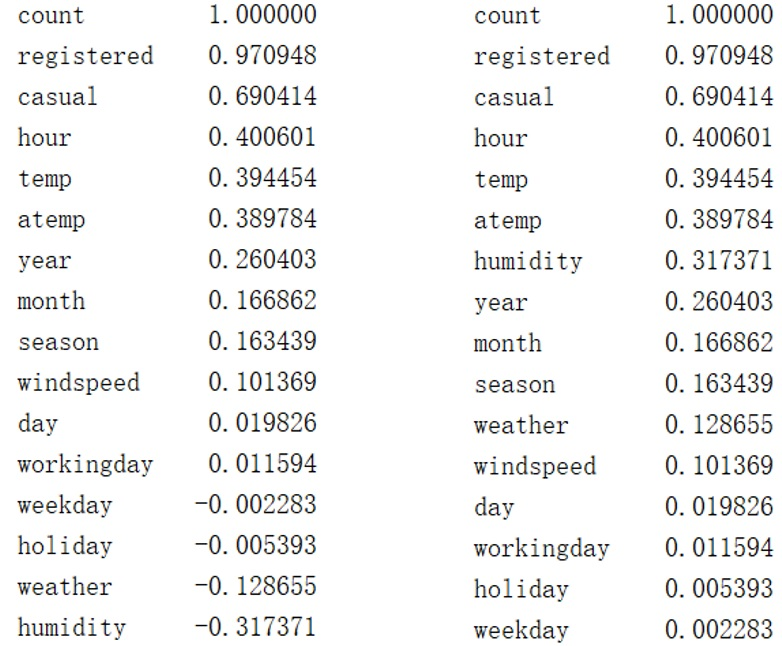从相关系数可以看出，天气（包括温度、湿度）对租借数存在明显影响，其中temp和atemp的意义及其与count的相关系数十分接近，因此可以只取atemp作为温度特征。此外，year、month、season等时间因素对count也存在明显影响，而holiday和weekday与count的相关系数极小。
为了更加直观地展现所有特征之间的影响，作相关系数热力图：

#2、作相关性分析的热力图

import matplotlib.pyplot
as plt

import seaborn
as sn

f,ax = plt.subplots(figsize=(
16,
16))

cmap = sn.cubehelix_palette(light=
1,as_cmap=
True)

sn.heatmap(correlation,annot=
True,center=
1,cmap=cmap,linewidths=
1,ax=ax)

sn.heatmap(correlation,vmax=
1,square=
True,annot=
True,linewidths=
1)

plt.show()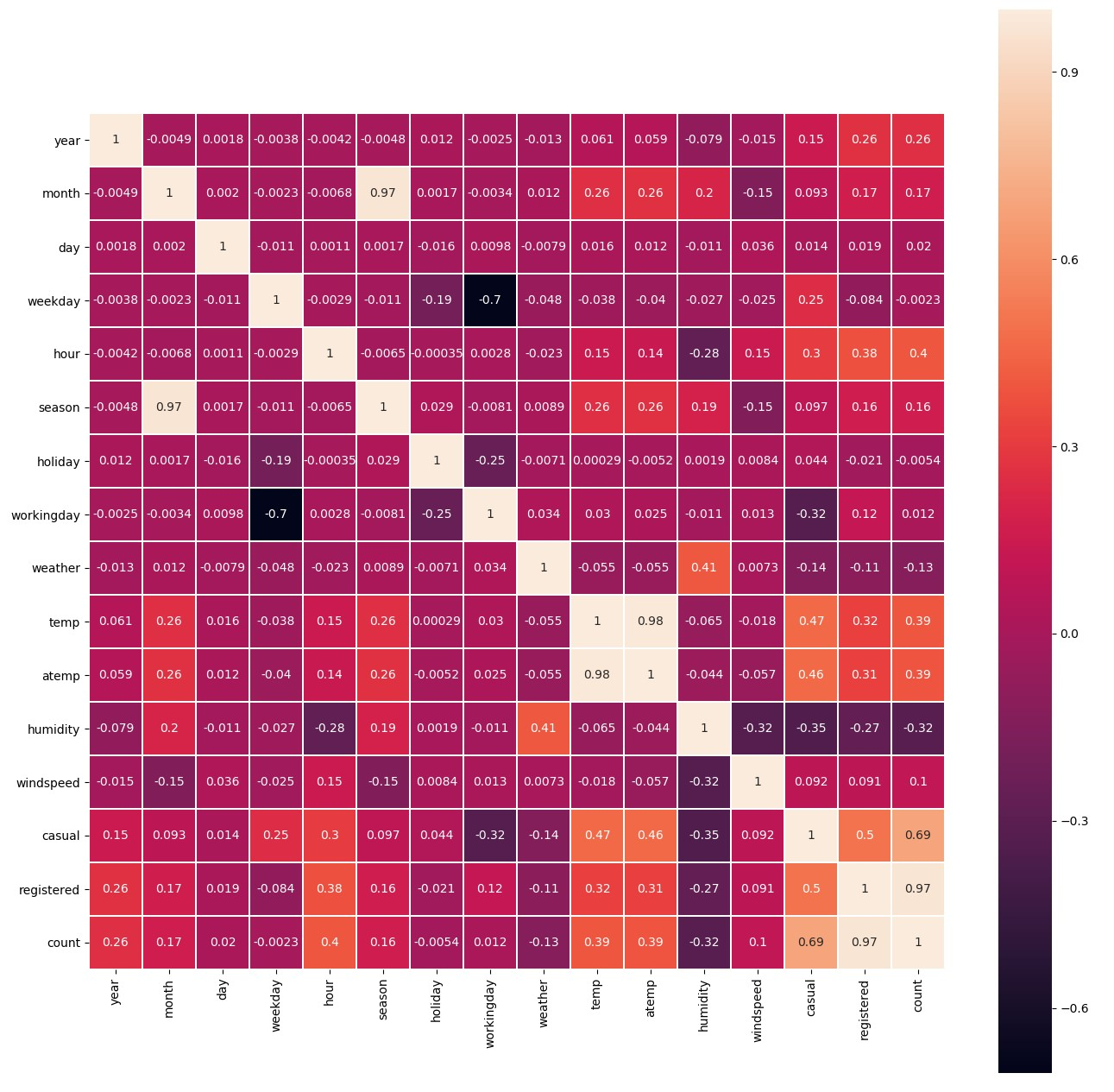接下来，深入分析各特征对租借数的影响规律，对每个特征进行可视化：

#3、每个特征对租借量的影响

#(1) 时间维度——年份

sn.boxplot(train[
‘year’],train[
‘count’])

plt.title(
“The influence of year”)

plt.show()

#(2) 时间维度——月份

sn.pointplot(train[
‘month’],train[
‘count’])

plt.title(
“The influence of month”)

plt.show()

#(3) 时间维度——季节

sn.boxplot(train[
‘season’],train[
‘count’])

plt.title(
“The influence of season”)

plt.show()

#(4) 时间维度——时间（小时）

sn.barplot(train[
‘hour’],train[
‘count’])

plt.title(
“The influence of hour”)

plt.show()

（1）年份对租借数的影响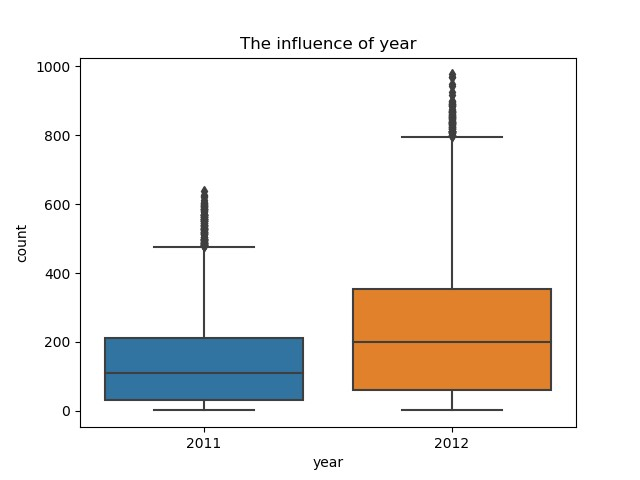2012年的租借数明显比2011年高，说明随着时间的推移，共享单车逐渐被更多的人熟悉和认可，使用者越来越多。
（2）月份对租借数的影响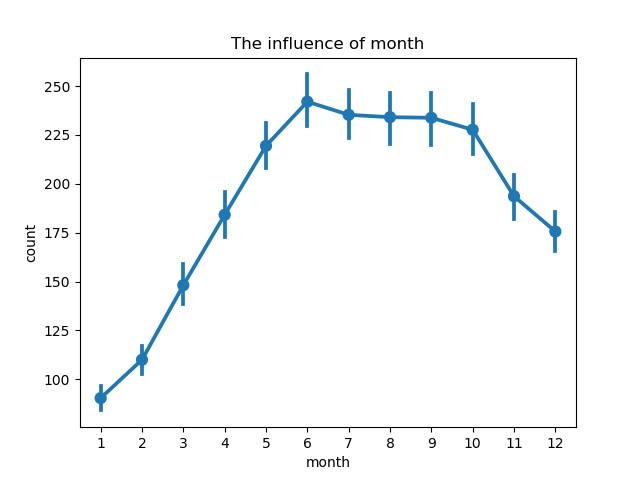月份对租借数影响显著，从1月份开始每月的租借数快速增加，到6月份达到顶峰，随后至10月缓慢降低，10月后急剧减少。这明显与季节有关。
（3）季节对租借数的影响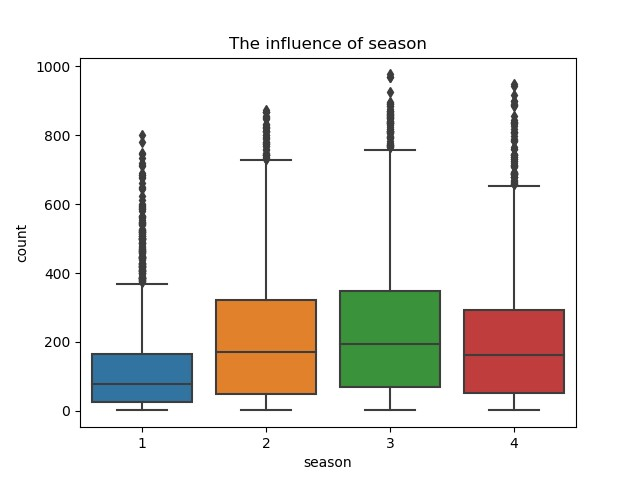通过各季度箱型图可以看出季节对租借数的影响符合预期：春季天气仍然寒冷，骑车人少；随着天气转暖，骑车人逐渐增多，并在秋季（天气最适宜时）达到顶峰；随后进入冬季，天气变冷，骑车人减少。
因为月份和季节对租借数的影响重合，且月份更加详细，因此在随后的建模过程中选取月份特征，删除季节特征。
（4）时间（小时）对租借数的影响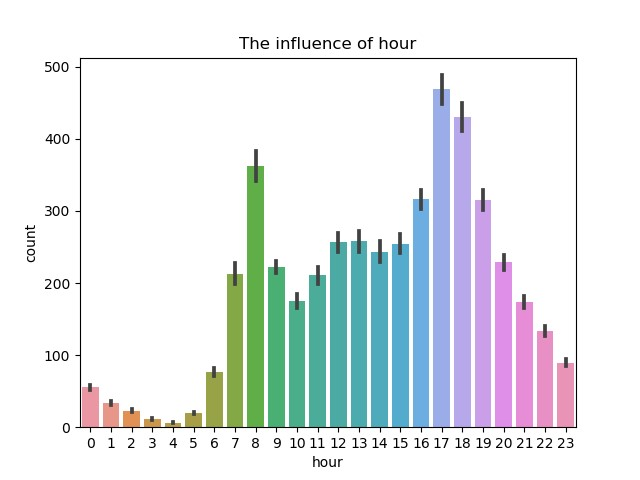从时间的分布上来看，每天有两个高峰期，分别是早上8点左右和下午17点左右，正好是工作日的上下班高峰期。而介于两者之间的白天时间变化规律不明显，可能与节假日有关，因此以此为基础需要考虑节假日和星期的影响。

#星期、节假日和工作日的影响

fig, axes = plt.subplots(
2,
1,figsize=(
16,
10))

ax1 = plt.subplot(
2,
1,
1)

sn.pointplot(train[
‘hour’],train[
‘count’],hue=train[
‘weekday’],ax=ax1)

ax1.set_title(
“The influence of hour (weekday)”)

ax2 = plt.subplot(
2,
2,
3)

sn.pointplot(train[
‘hour’],train[
‘count’],hue=train[
‘workingday’],ax=ax2)

ax2.set_title(
“The influence of hour (workingday)”)

ax3 = plt.subplot(
2,
2,
4)

sn.pointplot(train[
‘hour’],train[
‘count’],hue=train[
‘holiday’],ax=ax3)

ax3.set_title(
“The influence of hour (holiday)”)

plt.show()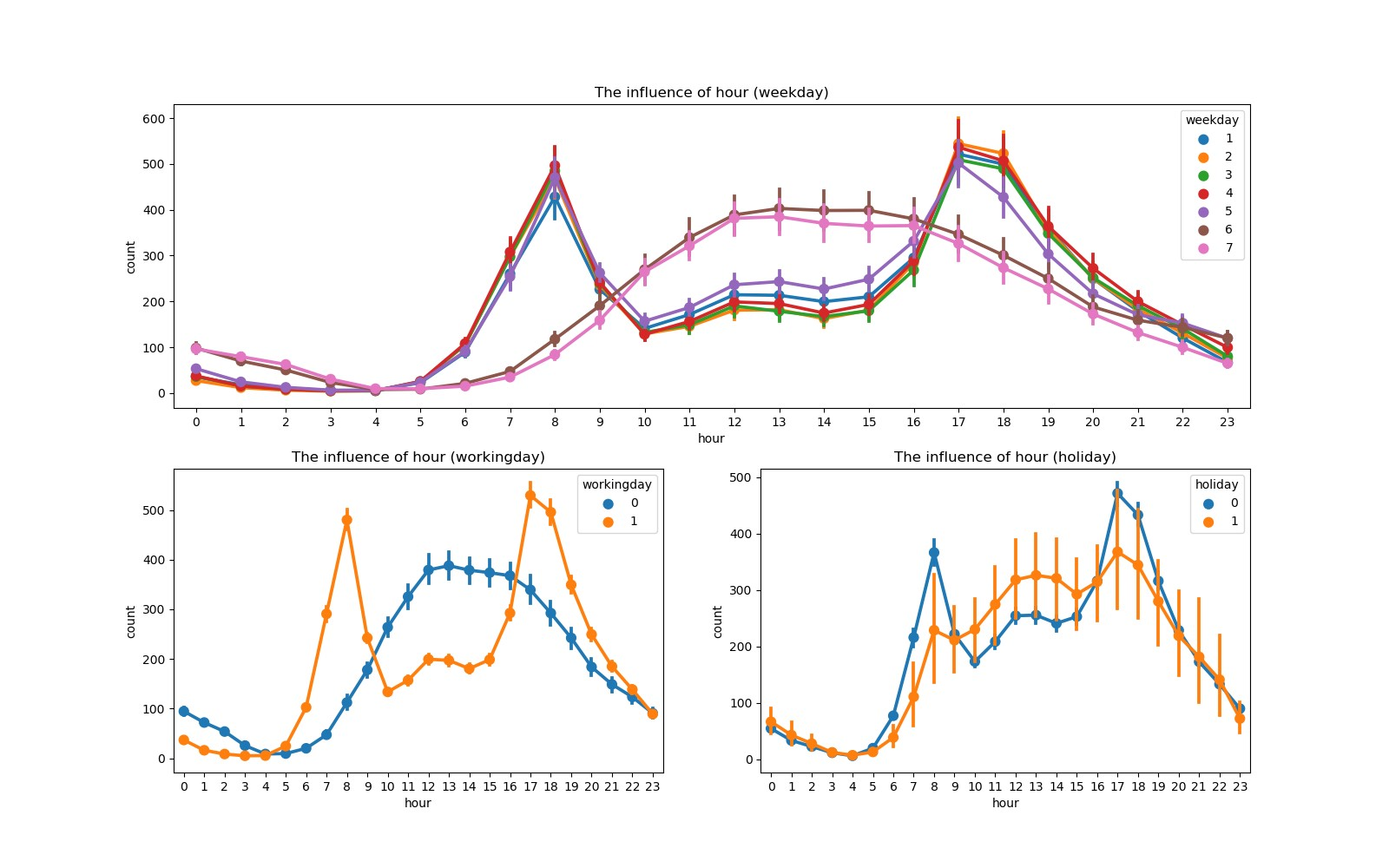可以看出，工作日早晚上班高峰期租借量高，其余时间租借量低；节假日中午及午后租借量较高，符合节假日人们出行用车的规律。
（5）天气对租借数的影响

#(5) 天气的影响

sn.boxplot(train[
‘weather’],train[
‘count’])

plt.title(
“The influence of weather”)

plt.show()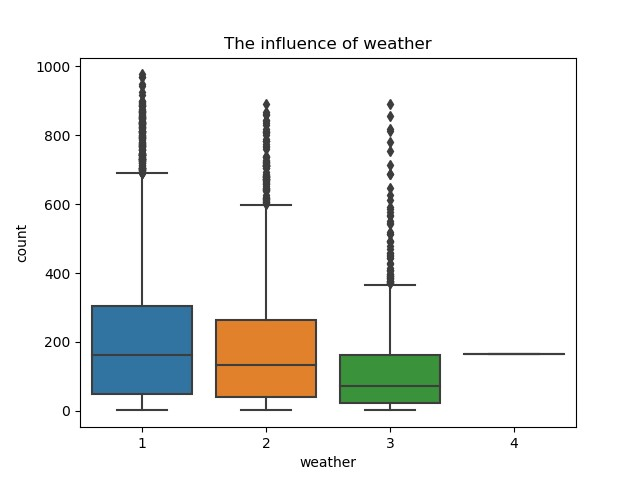（6）具体天气因素（温度、湿度和风速）的影响

#(6) 温度、湿度、风速的影响

cols = [
‘temp’,
‘atemp’,
‘humidity’,
‘windspeed’,
‘count’]

sn.pairplot(train[cols])

plt.show()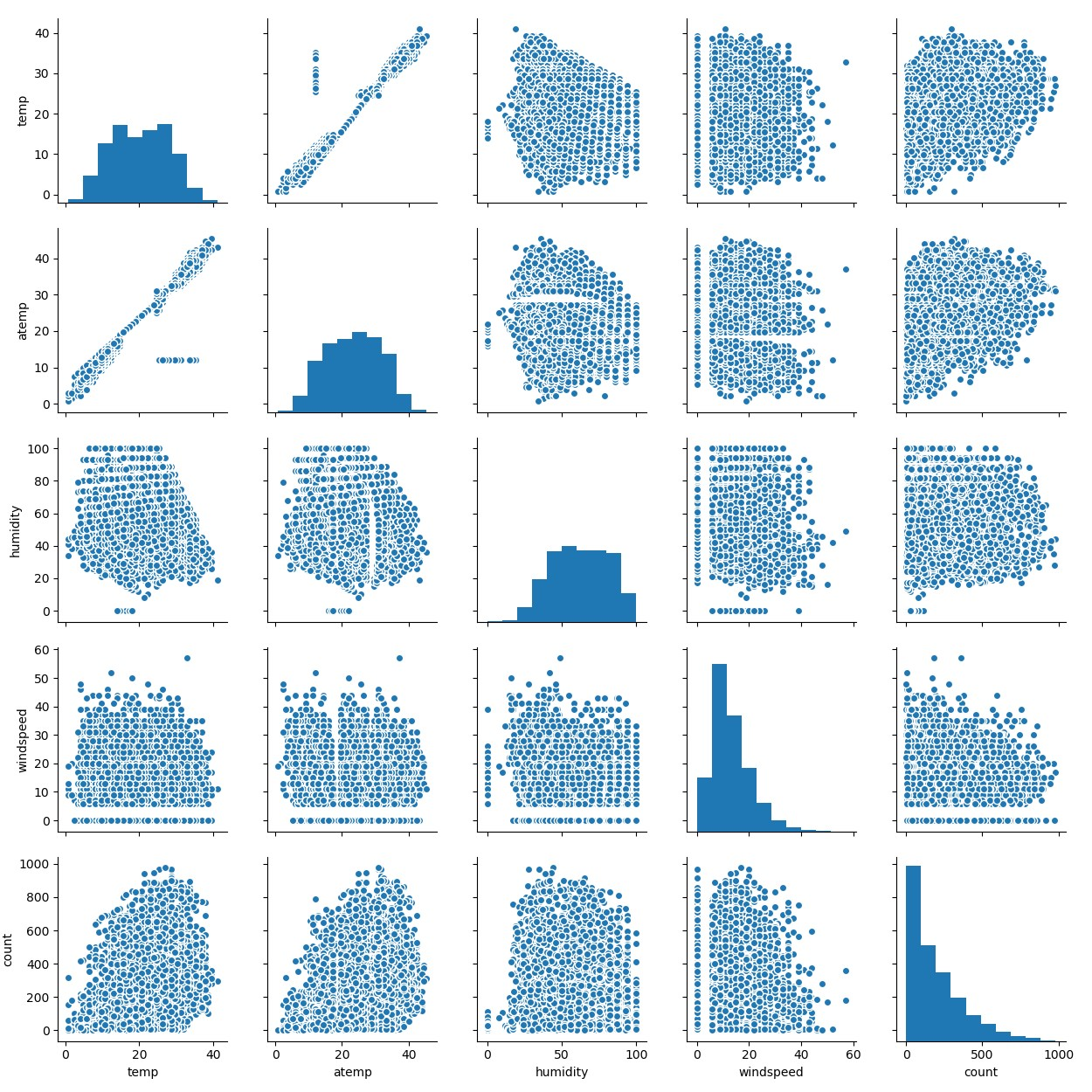作出多个连续变量之间的相关图，可以比较任意两个连续变量之间的关系。图中可以明显看出temp和atemp大致成线性关系，但也存在一组数据显著偏离线性相关趋势，可能与湿度和风速有关。因此，可以认为temp、humidity和windspeed三者共同决定了atemp，因此在后续建模过程中可以删除atemp特征。
进一步研究温度、湿度和风速对租借数的影响：

fig, axes = plt.subplots(
1,
3,figsize=(
24,
8))

ax1 = plt.subplot(
1,
3,
1)

ax2 = plt.subplot(
1,
3,
2)

ax3 = plt.subplot(
1,
3,
3)

sn.regplot(train[
‘temp’],train[
‘count’],ax=ax1)

sn.regplot(train[
‘humidity’],train[
‘count’],ax=ax2)

sn.regplot(train[
‘windspeed’],train[
‘count’],ax=ax3)

ax1.set_title(
“The influence of temperature”)

ax2.set_title(
“The influence of humidity”)

ax3.set_title(
“The influence of windspeed”)

plt.show()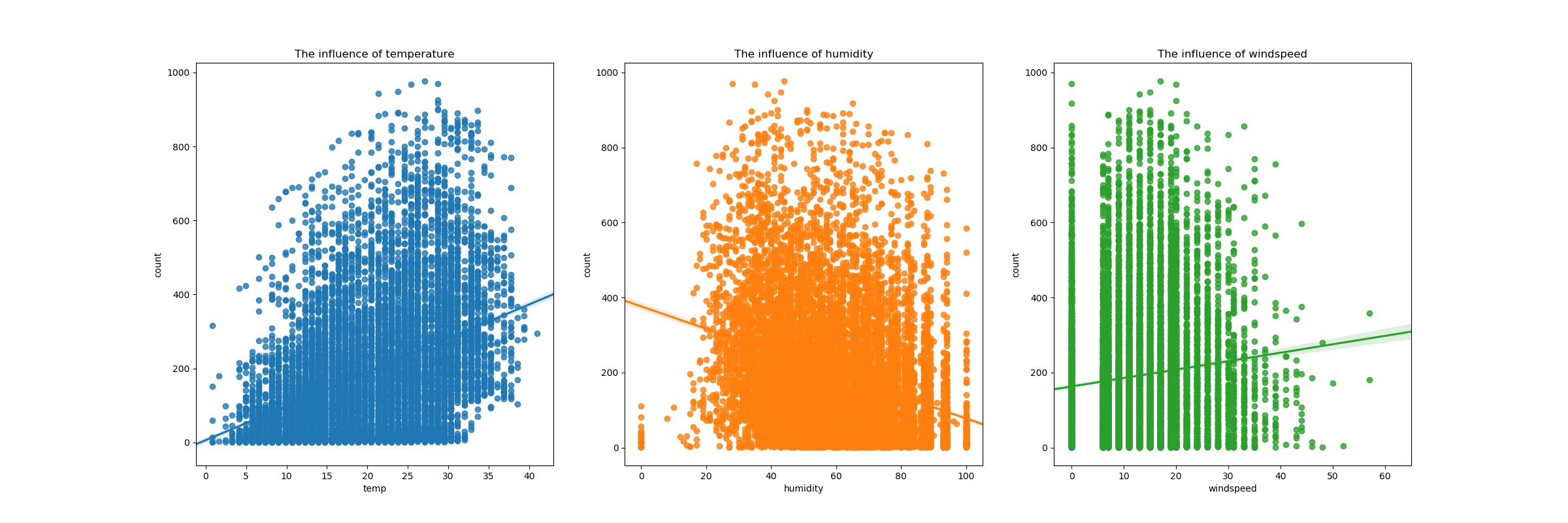虽然三种天气因素对租借数的影响比较分散，但可以明显看出温度和风速与租借数成正相关，湿度与租借数成负相关。
3.3 特征工程
综上所述，本项目提取特征year、month、hour、workingday、holiday、weather、temp、humidity和windspeed共9个特征预测租借总数。其中year、month、hour、workingday、holiday和weather为离散量，且由于workingday和holiday已经是二元属性，因此其余四个需要进行独热编码（one-hot）方式进行转换。

#特征工程

#所选取的特征：year、month、hour、workingday、holiday、weather、temp、humidity和windspeed

#(1) 删除不要的变量

data = data.drop([
‘day’,
‘weekday’,
‘season’,
‘atemp’,
‘casual’,
‘registered’],axis=
1)

#(2) 离散型变量（year、month、hour、weather）转换

column_trans = [
‘year’,
‘month’,
‘hour’,
‘weather’]

data = pd.get_dummies(data, columns=column_trans)

四、构建模型
接下来，需要对数据进行建模预测，分别采用三种典型集成学习模型（普通随机森林、极端随机森林模型和梯度提升树模型）、XGBoost模型和人工神经网络模型。此处均采用模型的默认参数或简单参数，如人工神经网络选用三层神经网络，每层包含神经元数量相同，且均为特征个数。

#机器学习

#1、特征向量化

col_trans = [
‘holiday’,
‘workingday’,
‘temp’,
‘humidity’,
‘windspeed’,

‘year_2011’,
‘year_2012’,
‘month_1’,
‘month_2’,
‘month_3’,
‘month_4’,

‘month_5’,
‘month_6’,
‘month_7’,
‘month_8’,
‘month_9’,
‘month_10’,

‘month_11’,
‘month_12’,
‘hour_0’,
‘hour_1’,
‘hour_2’,
‘hour_3’,

‘hour_4’,
‘hour_5’,
‘hour_6’,
‘hour_7’,
‘hour_8’,
‘hour_9’,
‘hour_10’,

‘hour_11’,
‘hour_12’,
‘hour_13’,
‘hour_14’,
‘hour_15’,
‘hour_16’,

‘hour_17’,
‘hour_18’,
‘hour_19’,
‘hour_20’,
‘hour_21’,
‘hour_22’,

‘hour_23’,
‘weather_1’,
‘weather_2’,
‘weather_3’,
‘weather_4’]

X_train = data[col_trans].iloc[:
10886]

X_test = data[col_trans].iloc[
10886:]

Y_train = data[
‘count’].iloc[:
10886]

from sklearn.feature_extraction
import DictVectorizer

vec = DictVectorizer(sparse=
False)

X_train = vec.fit_transform(X_train.to_dict(orient=
‘record’))

X_test = vec.fit_transform(X_test.to_dict(orient=
‘record’))

#分割训练数据

from sklearn.model_selection
import train_test_split

x_train, x_test, y_train, y_test = train_test_split(X_train, Y_train, test_size=
0.25, random_state=
40)

#2、建模预测，分别采用常规集成学习方法、XGBoost和神经网络三大类模型

from sklearn.ensemble
import RandomForestRegressor

from sklearn.ensemble
import ExtraTreesRegressor

from sklearn.ensemble

from xgboost
import XGBRegressor

from sklearn.neural_network
import MLPRegressor

from sklearn.metrics
import r2_score

#（1）集成学习方法——普通随机森林

rfr = RandomForestRegressor()

rfr.fit(x_train,y_train)

#print(rfr.fit(x_train,y_train))

rfr_y_predict = rfr.predict(x_test)

print(
“集成学习方法——普通随机森林回归模型的R方得分为：”,r2_score(y_test,rfr_y_predict))

#（2）集成学习方法——极端随机森林

etr = ExtraTreesRegressor()

etr.fit(x_train,y_train)

#print(etr.fit(x_train,y_train))

etr_y_predict = etr.predict(x_test)

print(
“集成学习方法——极端随机森林回归模型的R方得分为：”,r2_score(y_test,etr_y_predict))

#（3）集成学习方法——梯度提升树

gbr.fit(x_train,y_train)

#print(gbr.fit(x_train,y_train))

gbr_y_predict = gbr.predict(x_test)

print(
“集成学习方法——梯度提升树回归模型的R方得分为：”,r2_score(y_test,gbr_y_predict))

#（4） XGBoost回归模型

xgbr = XGBRegressor()

xgbr.fit(x_train,y_train)

#print(xgbr.fit(x_train,y_train))

xgbr_y_predict = xgbr.predict(x_test)

print(
“XGBoost回归模型的R方得分为：”,r2_score(y_test,xgbr_y_predict))

#（5） 神经网络回归模型

mlp = MLPRegressor(hidden_layer_sizes=(
47,
47,
47),max_iter=
500)

mlp.fit(x_train,y_train)

mlp_y_predict = mlp.predict(x_test)

print(
“神经网络回归模型的R方得分为：”,r2_score(y_test,mlp_y_predict))

最终模型预测能力如下：可以看出，默认配置的随机森林模型和简单参数的人工神经网络R方得分较高。分别采用极端随机森林模型和人工神经网络预测测试数据，上传至Kaggle评分，两者结果相似，其中极端随机森林模型的效果略好，结果如下：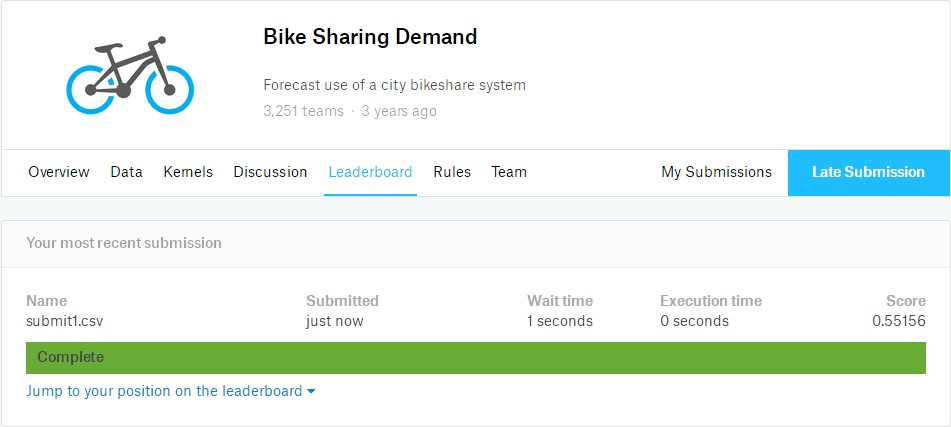本项目的得分为均方根对数误差（RMSLE），值越小越好。该比赛官方记录最好得分为0.33756。因此，该模型还有待提高，主要是对模型进行调参。每种机器学习模型均有若干参数，需理解每个参数的意义和作用，采用适当方法进行调参，以期获得最佳参数，提高预测准确率。
下面以极端随机森林模型为例，进行调参。需要了解模型参数及调参步骤，该部分内容已在另一篇原创文章中详细阐述：https://blog.csdn.net/Caesar1993_Wang/article/details/80337103
最终通过调参，获得最佳参数（random_state=27，n_estimators=41，max_depth=38，max_features=14），预测结果也有所提高：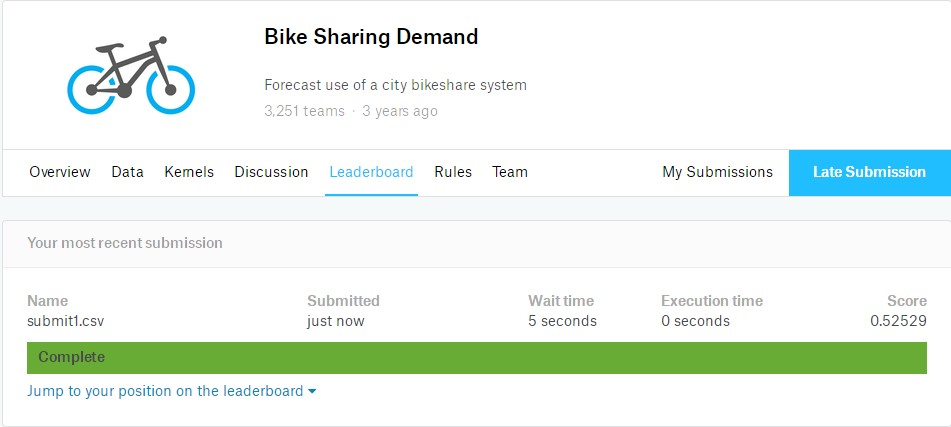本项目基本流程已经结束，但还有许多可以改进的地方：分别预测注册和未注册的租借数；对连续性特征量进行归一化；使用神经网络对预测模型的参数进行大规模调参。
展开全文•文档
• 共享单车是指企业在校园、地铁站点、公交站点、居民区、商业区、公共服务区等提供自行车单车共享服务，是一种分时租赁模式，是一种新型绿色环保共享经济。 共享单车系统是一种租赁自行车的方法，注册会员、租车、还...
一、项目背景 共享单车是指企业在校园、地铁站点、公交站点、居民区、商业区、公共服务区等提供自行车单车共享服务，是一种分时租赁模式，是一种新型绿色环保共享经济。
共享单车系统是一种租赁自行车的方法，注册会员、租车、还车都将通过城市中的站点网络自动完成。使用共享单车，可以注册会员，存入一定金额用于使用结束后自动扣费结算；也可以不注册会员，临时使用后通过其提供的支付方式结算费用。人们通过下载APP使用这个系统进行扫码开锁取车，然后从取车地骑到自己的目的地后停放锁车归还，系统自动按实际使用时长计费。
共享单车是近年来流行起来的新兴产业，发展具有重要价值通过对租车数据进行统计以及可视化分析，对单车运营维护团队提出改善性意见
二、项目分析具体步骤
#导入工具包
import datetime
import numpy as np
import pandas as pd
import seaborn as sns
import matplotlib.pyplot 
展开全文• （原本这个项目的目的是预测华盛顿地区的共享单车的租赁需求量，在这里我们主要任务是将共享单车的数据进行可视化分析，不着重选择合适的机器学习模型来预测需求量。）三、理解数据：import pandas as pd import n.....
• Python数据分析实战项目-共享单车有效的解决了“走路累，公交挤，开车堵，打车贵”的苦恼。一夜之间，北上广深、甚至部分二线城市，共享单车大街小巷随处可见。
• 文章目录摩拜共享单车数据分析项目报告项目背景数据探索数据挖掘数据分析时间维度空间维度用户维度 摩拜共享单车数据分析项目报告 项目背景 随着智能手机的普及和手机用户的激增，共享单车作为城市交通系统的一个...
• 项目背景：提供两年的每...本次共享单车数据分析项目数据源于Kaggle [https://www.kaggle.com/c/bike-sharing-demand/data]。 1.2 载入数据 // 载入工具包 import numpy as np import pandas as pd import matplotlib.
• 美国共享单车数据分析数据说明数据清洗空值删除数据类型转化统计值概览异常数据数据筛选新增小时、周、月纬度探索性分析数据概览数据异常分析bike_plan与会员关系小时、周、月纬度分析高峰时间骑行数据订阅会员分析...python
• 数据：2017年5月两周内，北京40余万辆摩拜单车被30余万摩拜用户的使用情况(该开源数据来源于2017年摩拜算法挑战赛)。数据包含300余万条出行记录数据，每条的数据包含了订单号(orderid )、用户编号(userid )、单车...
• alkingData-共享单车人群分析报告.pdf
• 前几天是小文人生中一个非常...这是情（zhuang）调（bi）懂不懂… 那天之后，小文就想做一个关于共享单车分析，上kaggle看见有一个数据集---bike sharing，那是美国华盛顿共享单车数据，也是非常适合练手的一个...kaggle R语言
• ## 共享单车竞品分析

千次阅读 2019-05-16 12:08:03...